Question

Which of the following aqueous solutions are good buffer systems? .

__0.10 M sodium hydroxide + 0.27 M sodium chloride.

__0.28 M ammonia + 0.34 M ammonium nitrate.

__0.25 M perchloric acid + 0.25 M sodium perchlorate.

__0.32 M sodium iodide + 0.23 M potassium iodide.

__0.15 M hydrocyanic acid + 0.16 M hydroiodic acid.

acidic buffer is formed by weak acid and its conjugate base

basic buffer is formed by weak base and its conjugate acid

1)

NaOH is strong base and NaCl is neutral salt.

They will not form buffer.

2)

NH3 is weak base and NH4+ is its conjugate acid.

They will form basic buffer

3)

HClO4 is strong acid and NaClO4 is neutral salt.

They will not form buffer.

4)

NaI and KI are both neutral salt.

They will not form buffer.

5)

HCN and HI are both weak acid

They will not form buffer.

False

True

False

False

False

#### Earn Coins

Coins can be redeemed for fabulous gifts.

Similar Homework Help Questions
• ### Which of the following aqueous solutions are good buffer systems? . 0.27 M nitric acid +...

Which of the following aqueous solutions are good buffer systems? . 0.27 M nitric acid + 0.20 M sodium nitrate 0.30 M ammonia + 0.30 M sodium hydroxide 0.34 M barium iodide + 0.28 M calcium iodide 0.19 M potassium hydroxide + 0.25 M potassium chloride 0.21 M hydrocyanic acid + 0.18 M potassium cyanide 0.16 M hydrocyanic acid + 0.14 M potassium cyanide 0.20 M potassium acetate + 0.28 M acetic acid 0.21 M perchloric acid + 0.20 M...

• ### Which of the following aqueous solutions are good buffer systems? 0.18 M hydrocyanic acid + 0.19...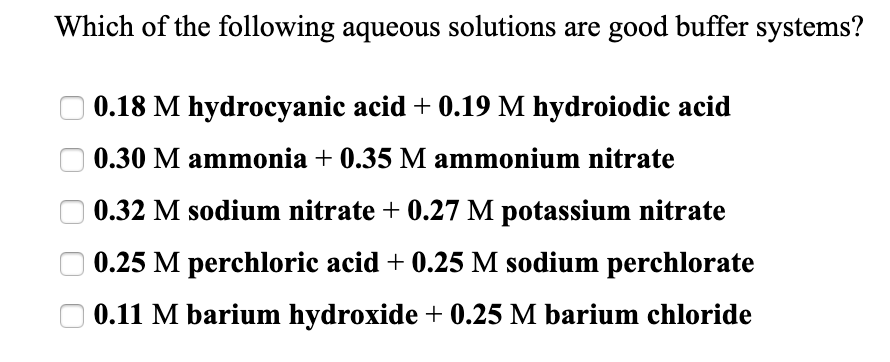Which of the following aqueous solutions are good buffer systems? 0.18 M hydrocyanic acid + 0.19 M hydroiodic acid 0.30 M ammonia + 0.35 M ammonium nitrate 0.32 M sodium nitrate + 0.27 M potassium nitrate 0.25 M perchloric acid + 0.25 M sodium perchlorate 0.11 M barium hydroxide + 0.25 M barium chloride

• ### Which of the following aqueous solutions are good buffer systems? 0.10 M acetic acid + 0.11...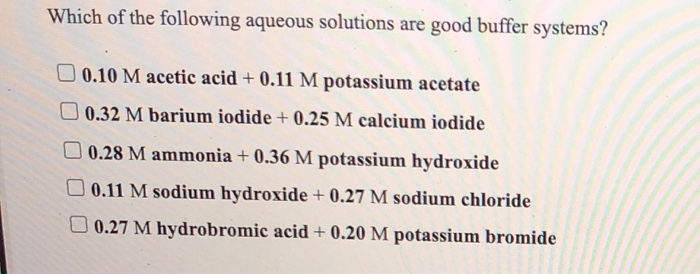Which of the following aqueous solutions are good buffer systems? 0.10 M acetic acid + 0.11 M potassium acetate 0.32 M barium iodide + 0.25 M calcium iodide 0.28 M ammonia + 0.36 M potassium hydroxide 0.11 M sodium hydroxide + 0.27 M sodium chloride 0.27 M hydrobromic acid + 0.20 M potassium bromide Which of the following aqueous solutions are good buffer systems? 0.37 Macetic acid + 0.21 M sodium acetate 0.14 M sodium hydroxide + 0.29 M sodium...

• ### Which of the following aqueous solutions are good buffer systems? 0.25 M perchloric acid + 0.23...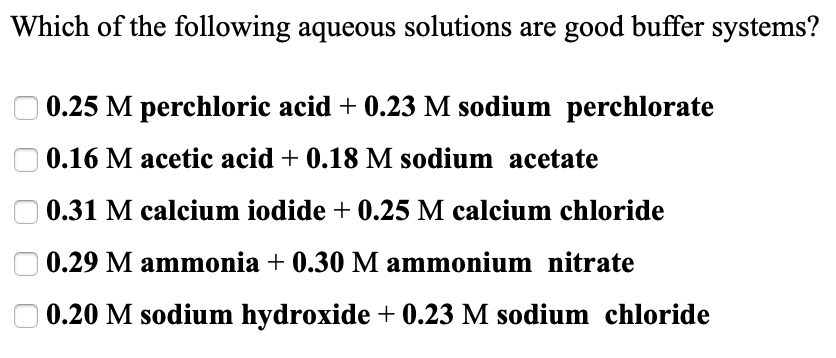Which of the following aqueous solutions are good buffer systems? 0.25 M perchloric acid + 0.23 M sodium perchlorate 0.16 M acetic acid + 0.18 M sodium acetate 0.31 M calcium iodide + 0.25 M calcium chloride 0.29 M ammonia + 0.30 M ammonium nitrate 0.20 M sodium hydroxide + 0.23 M sodium chloride

• ### Which of the following aqueous solutions are good buffer systems? 0.31 M ammonia +0.38 M sodium...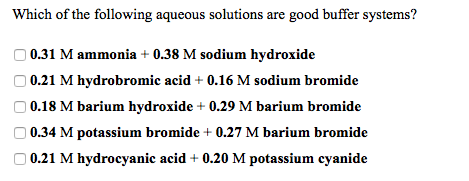Which of the following aqueous solutions are good buffer systems? 0.31 M ammonia +0.38 M sodium hydroxide 0.21 M hydrobromic acid + 0.16 M sodium bromide 0.18 M barium hydroxide + 0.29 M barium bromide 0.34 M potassium bromide + 0.27 M barium bromide 0.21 M hydrocyanic acid + 0.20 M potassium cyanide Which of the following aqueous solutions are good buffer systems? 0.21 M acetic acid + 0.11 M hydrochloric acid 0.11 M sodium hydroxide + 0.24 M sodium...

• ### Which of the following aqueous solutions are good buffer systems? 0.29 M perchloric acid + 0.16...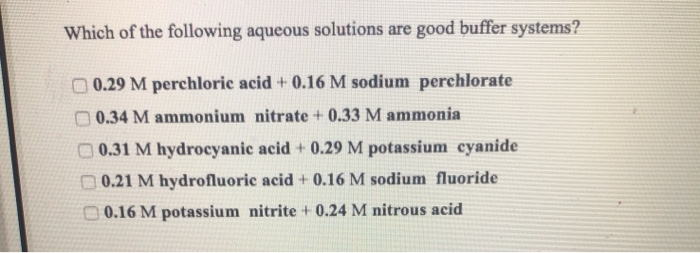Which of the following aqueous solutions are good buffer systems? 0.29 M perchloric acid + 0.16 M sodium perchlorate 0.34 M ammonium nitrate + 0.33 M ammonia 0.31 M hydrocyanic acid + 0.29 M potassium cyanide 0.21 M hydrofluoric acid + 0.16 M sodium fluoride 0.16 M potassium nitrite + 0.24 M nitrous acid

• ### Which of the following aqueous solutions are good buffer systems? 0.16 M potassium hydroxide + 0.29...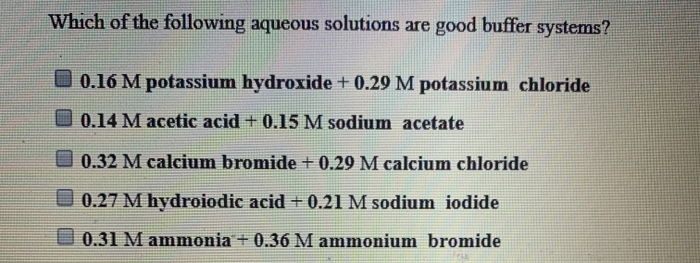Which of the following aqueous solutions are good buffer systems? 0.16 M potassium hydroxide + 0.29 M potassium chloride 0.14 M acetic acid + 0.15 M sodium acetate 0.32 M calcium bromide + 0.29 M calcium chloride U 0.27 M hydroiodic acid + 0.21 M sodium iodide U 0.31 M ammonia + 0.36 M ammonium bromide

• ### Which of the following aqueous solutions are good buffer systems? 0.31 M hydrocyanic acid0.27 M sodium...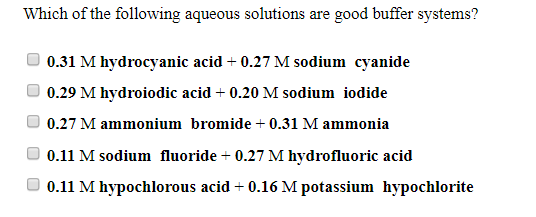Which of the following aqueous solutions are good buffer systems? 0.31 M hydrocyanic acid0.27 M sodium cyanide 0.29 M hydroiodic acid 0.20 M sodium iodide 0.27 M ammonium bromide + 0.31 M ammonia O 0.11 M sodium fluoride-0.27 M hydrofluoric acid 0.11 M hypochlorous acid 0.16 M potassium hypochlorite

• ### Which of the following aqueous solutions are good buffer systems?

Which of the following aqueous solutions are good buffer systems? 0.12 M calcium hydroxide + 0.22 M calcium chloride 0.21 M hydroiodic acid + 0.25 M sodium iodide 0.19 M nitrous acid + 0.14 M potassium nitrite 0.37 M hypochlorous acid + 0.27 M potassium hypochlorite 0.34 Mammonium nitrate + 0.33 Mammonia

• ### Which of the following aqueous solutions are good buffer systems? 0.11 M potassium hydroxide +0.20 M...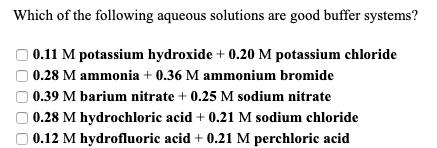Which of the following aqueous solutions are good buffer systems? 0.11 M potassium hydroxide +0.20 M potassium chloride 0.28 M ammonia + 0.36 M ammonium bromide 0.39 M barium nitrate + 0.25 M sodium nitrate 0.28 M hydrochloric acid + 0.21 M sodium chloride 0.12 M hydrofluoric acid + 0.21 M perchloric acid